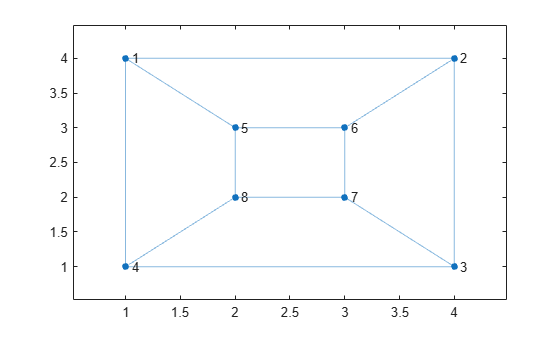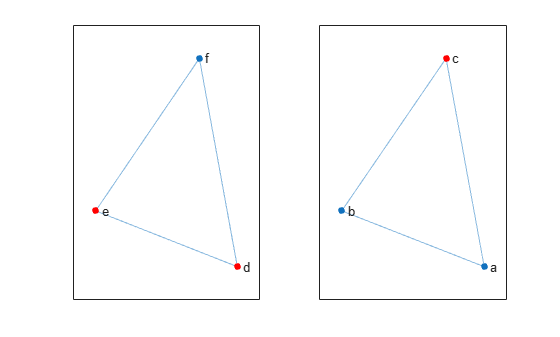Documentation

### This is machine translation

Mouseover text to see original. Click the button below to return to the English version of the page.

# isisomorphic

Determine whether two graphs are isomorphic

## Syntax

``tf = isisomorphic(G1,G2)``
``tf = isisomorphic(G1,G2,Name,Value)``

## Description

example

````tf = isisomorphic(G1,G2)` returns logical `1` (`true`) if a graph isomorphism exists between graphs `G1` and `G2`; otherwise, it returns logical `0` (`false`).```

example

````tf = isisomorphic(G1,G2,Name,Value)` specifies additional options with one or more name-value pair arguments. For example, you can specify `'NodeVariables'` and a list of node variables to indicate that the isomorphism must preserve these variables to be valid.```

## Examples

collapse all

Create and plot two directed graphs, and then determine if they are isomorphic.

```G1 = digraph([1 1 1 2 3 4],[2 3 4 4 4 1]); G2 = digraph([3 3 3 2 1 4],[1 4 2 3 2 2]); subplot(1,2,1) plot(G1) subplot(1,2,2) plot(G2)````isisomorphic(G1,G2)`
```ans = logical 1 ```

Create and plot two graphs, `G1` and `G2`.

```G1 = graph([1 1 1 2 2 3 3 4 5 5 7 7],[2 4 5 3 6 4 7 8 6 8 6 8]); plot(G1,'XData',[1 4 4 1 2 3 3 2],'YData',[4 4 1 1 3 3 2 2])``````G2 = graph({'a' 'a' 'a' 'b' 'b' 'b' 'c' 'c' 'c' 'd' 'd' 'd'}, ... {'g' 'h' 'i' 'g' 'h' 'j' 'g' 'i' 'j' 'h' 'i' 'j'}); plot(G2,'XData',[1 2 2 2 1 2 1 1],'YData',[4 4 3 2 3 1 2 1])```Determine whether an isomorphism exists for `G1` and `G2`. The result indicates that the graphs are structurally the same despite their different labels and layouts.

`tf = isisomorphic(G1,G2)`
```tf = logical 1 ```

Use two different comparisons to determine if there is an isomorphism relation between two graphs. One of the comparisons preserves a node property, while the other ignores it.

Create two similar graphs. Add a node property `Color` to each of the graphs.

```G1 = graph({'d' 'e' 'f'},{'e' 'f' 'd'}); G1.Nodes.Color = {'red' 'red' 'blue'}'; G2 = graph({'a' 'b' 'c'},{'b' 'c' 'a'}); G2.Nodes.Color = {'blue' 'blue' 'red'}';```

Plot the graphs side-by-side in the same figure. Color the nodes red that have `Color = 'red'`.

```subplot(1,2,1) p1 = plot(G1); highlight(p1,{'d' 'e'},'NodeColor','r') subplot(1,2,2) p2 = plot(G2); highlight(p2,'c','NodeColor','r')```Determine if the graphs are isomorphic, ignoring the `Color` property.

`tf = isisomorphic(G1,G2)`
```tf = logical 1 ```

Determine if the graphs are isomorphic and preserve the value of the `Color` property in the comparison. In this case, there is no isomorphism since the `Color` property of each graph contains different numbers of `'red'` and `'blue'` values.

`tf = isisomorphic(G1,G2,'NodeVariables','Color')`
```tf = logical 0 ```

## Input Arguments

collapse all

Input graphs, specified as separate arguments of `graph` or `digraph` objects. Use `graph` to create an undirected graph or `digraph` to create a directed graph.

`G1` and `G2` must be both `graph` objects or both `digraph` objects.

Example: `G1 = graph(1,2)`

Example: `G1 = digraph([1 2],[2 3])`

### Name-Value Pair Arguments

Specify optional comma-separated pairs of `Name,Value` arguments. `Name` is the argument name and `Value` is the corresponding value. `Name` must appear inside quotes. You can specify several name and value pair arguments in any order as `Name1,Value1,...,NameN,ValueN`.

Example: ```tf = isisomorphic(G1,G2,'NodeVariables',{'Var1' 'Var2'})```

Edge variables to preserve, specified as the comma-separated pair consisting of `'EdgeVariables'` and a character vector, string scalar, cell array of character vectors, or string array. Use this option to specify one or more edge variables that are in both `G1.Edges` and `G2.Edges`. The isomorphism comparison must preserve the specified edge variables in order to be valid. For multigraphs with multiple edges between the same two nodes, the ordering of the edge variables for the same node pair is irrelevant.

Data Types: `char` | `string` | `cell`

Node variables to preserve, specified as the comma-separated pair consisting of `'NodeVariables'` and a character vector, string scalar, cell array of character vectors, or string array. Use this option to specify one or more node variables that are in both `G1.Nodes` and `G2.Nodes`. The isomorphism comparison must preserve the specified node variables in order to be valid.

Data Types: `char` | `string` | `cell`

collapse all

### Graph Isomorphism

Two graphs, `G1` and `G2`, are isomorphic if there exists a permutation of the nodes `P` such that `reordernodes(G2,P)` has the same structure as `G1`.

Two graphs that are isomorphic have similar structure. For example, if a graph contains one cycle, then all graphs isomorphic to that graph also contain one cycle.